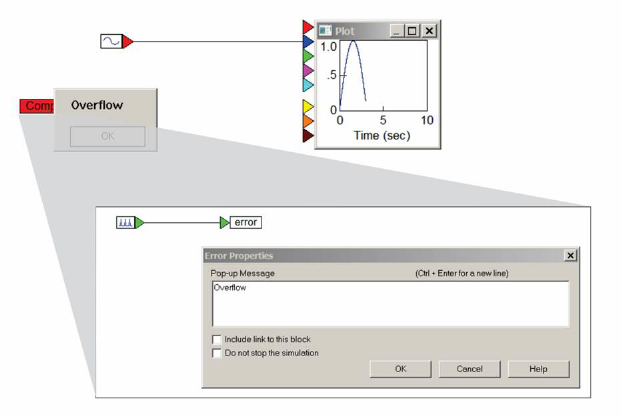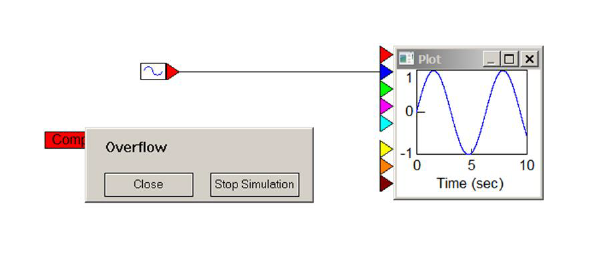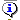## -XBlock Category: Arithmetic

Inputs: Real, complex, or fixed-point scalars, or vectors or matrices.

Description: The -X block negates the input signal.Label: Indicates a user-defined block label that appears when View > Block Labels is activated.

#### Examples

1. Negation of a scalar

Consider the equation y(t) = - sin(t) realized as:A ramp block is used to access simulation time t, a sin block generates sin(t), and a -X block converts sin(t) to -sin(t). Both sin(t) and y(t) are plotted for comparison.

2. Negation of a vector

Consider the equation:

z = -x

where x = [-1  5.6  4] realized as:A scalarToVector block creates a three-element vector from the constant values -1, 5.6, and 4. When the simulation runs, the -X block performs an element-by-element negate operation on the incoming vector.

3. Negation of a matrix

Consider the equation:

Z = -X

whererealized as:When the simulation runs, the -X block performs an element-by-element negate operation on the incoming matrix.